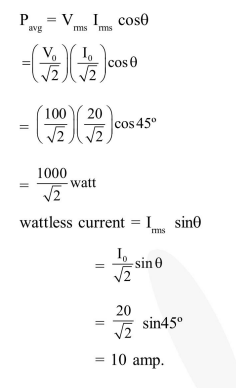Deepak Scored 45->99%ile with Bounce Back Crack Course. You can do it too!

# In an a. c. circuit, the instantaneous e.m.f.

Question:

In an a. c. circuit, the instantaneous e.m.f. and current are given by e $=100 \sin 30 \mathrm{t} \mathrm{i}=20 \sin \left(30 \mathrm{t}-\frac{\pi}{4}\right)$ In one cycle of a.c., the average power consumed by the circuit and the wattless current are, respectively.

1. $\frac{1000}{\sqrt{2}}, 10$

2. $\frac{50}{\sqrt{2}}, 0$

3.  50,0

4. 50,10

Correct Option: 1

Solution: